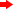With the recent publication of PHYSICS IS... there are now three Ask the Physicist books! Click on the book images below for information on the content of the books and for information on ordering.QUESTION:
In lifting an object to a higher level directly over its original location, the energy I expend increases potential energy. But, does some of the energy used in lifting it also go to accelerating it to higher rotational velocities as the circumference of its "orbit" increases as it is raised over its original position? Does this add kinetic energy and mass to the object whereas increasing potential energy does not?ANSWER:
(Preface: all my calculations below assume that the height lifted is much smaller than the radius of the earth. I also neglect the change in the gravitational force over the distance the mass is lifted. Also, to simplify things, all my calculations are at the equator.) Yes, work is done to increase the kinetic energy. As viewed from an inertial frame, watching the mass M get lifted to h, I estimate that the kinetic energy changes by
ΔK≈2hKinitial/R=MhRω2 where R=6.4x106 m is the radius of the earth and ω=7.3x10-5 s-1 is the angular velocity of the earth. For example, lifting 1 kg a height of 1 m requires 0.03 J of work to increase the kinetic energy. But wait a minute! Once we acknowledge that the earth is rotating, we have to recognize that the mass, being in a circular orbit, has a centripetal acceleration ac=2 and therefore the net force on M is Mg-MRω2. Therefore, the net work done is W≈(Mg-MRω2)h+MhRω2=Mgh.